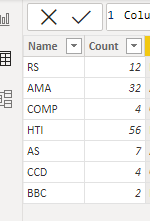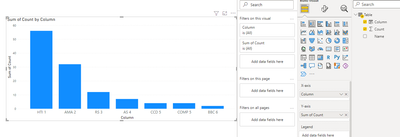cancel
Showing results for
Did you mean:Helper I

## Bar Chart ranking

Hi,

Is it possible to add the rank under each bar? For example, 1 under Rs, 2 under AMA. Thank you.1 ACCEPTED SOLUTIONCommunity Support

Hi , @gdxy

According to your description , you want to display the rank number in the x-axis of the bar chart. Right?

The X-axis of the bar chart shows the names of the fields we placed, and if we want to show the corresponding rankings additionally, we need to create a new column to represent the relationship between the name and the rank.

(1)This is my test data:(2)We can create a calculated column :

``Column = [Name]  & UNICHAR(10)&  RANKX(ALL('Table'[Name]) , CALCULATE(SUM('Table'[Count]) , ALLEXCEPT('Table','Table'[Name])),,,Dense)``(3)Then we use this [Column] to replace the x-axis field in the visual.There is no way to achieve line breaks in bar charts.The result is as follows:Best Regards,

Aniya Zhang

If this post helps, then please consider Accept it as the solution to help the other members find it more quickly

2 REPLIES 2Community Support

Hi , @gdxy

According to your description , you want to display the rank number in the x-axis of the bar chart. Right?

The X-axis of the bar chart shows the names of the fields we placed, and if we want to show the corresponding rankings additionally, we need to create a new column to represent the relationship between the name and the rank.

(1)This is my test data:(2)We can create a calculated column :

``Column = [Name]  & UNICHAR(10)&  RANKX(ALL('Table'[Name]) , CALCULATE(SUM('Table'[Count]) , ALLEXCEPT('Table','Table'[Name])),,,Dense)``(3)Then we use this [Column] to replace the x-axis field in the visual.There is no way to achieve line breaks in bar charts.The result is as follows:Best Regards,

Aniya Zhang

If this post helps, then please consider Accept it as the solution to help the other members find it more quicklyHelper I

Thank you for your answer, it inspired me to make what I would like to show.